Next: Exercises Up: Calculus of Variations Previous: Conditional Variation

# Multi-Function Variation

Suppose that we wish to maximize or minimize the functional(E.33)

Here, the integrandis now a functional of the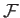independent functions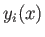, for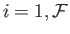. A fairly straightforward extension of the analysis in Section E.2 yieldsseparate Euler-Lagrange equations,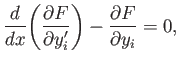(E.34)

for, which determine thefunctions. Ifdoes not explicitly depend on the functionthen the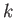th Euler-Lagrange equation simplifies to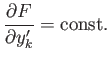(E.35)

Likewise, ifdoes not explicitly depend onthen allEuler-Lagrange equations simplify to(E.36)

for.

Richard Fitzpatrick 2016-03-31